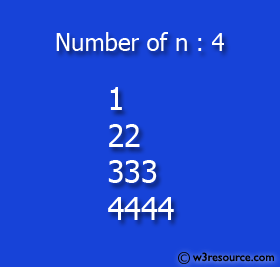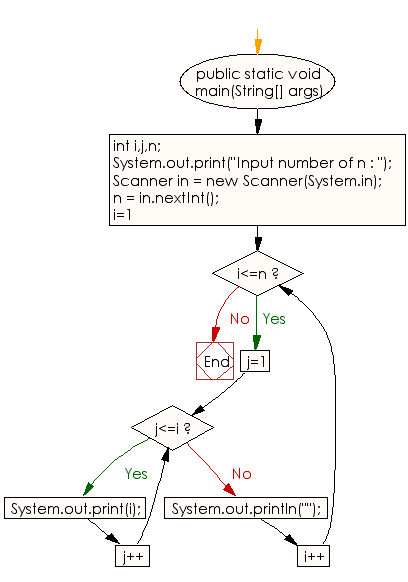﻿ Java - Print a pattern like right angle triangle

# Java: Print a pattern like right angle triangle

## Java Conditional Statement: Exercise-17 with Solution

Write a program in Java to make such a pattern like a right angle triangle with a number which repeats a number in a row.
The pattern is as follows :

```1
22
333
4444
```

Test Data
Input number of n : 4

Pictorial Presentation:Sample Solution:

Java Code:

``````import java.util.Scanner;
public class Exercise17 {

public static void main(String[] args)

{
int i,j,n;

System.out.print("Input number of n : ");
Scanner in = new Scanner(System.in);
n = in.nextInt();

for(i=1;i<=n;i++)
{
for(j=1;j<=i;j++)
System.out.print(i);
System.out.println("");
}
}
}
```
```

Sample Output:

```Input number of n : 4
1
22
333
4444

```

Flowchart:Java Code Editor:

What is the difficulty level of this exercise?

Test your Programming skills with w3resource's quiz.

﻿

## Java: Tips of the Day

IsPowerOfTwo

Checks if a value is positive power of two.

To understand how it works let's assume we made a call IsPowerOfTwo(4).

As value is greater than 0, so right side of the && operator will be evaluated.

The result of (~value + 1) is equal to value itself. ~100 + 001 => 011 + 001 => 100. This is equal to value.

The result of (value & value) is value. 100 & 100 => 100.

This will value the expression to true as value is equal to value.

```public static boolean isPowerOfTwo(final int value) {
return value > 0 && ((value & (~value + 1)) == value);
}
```

Ref: https://bit.ly/3sA5d4I

We are closing our Disqus commenting system for some maintenanace issues. You may write to us at reach[at]yahoo[dot]com or visit us at Facebook